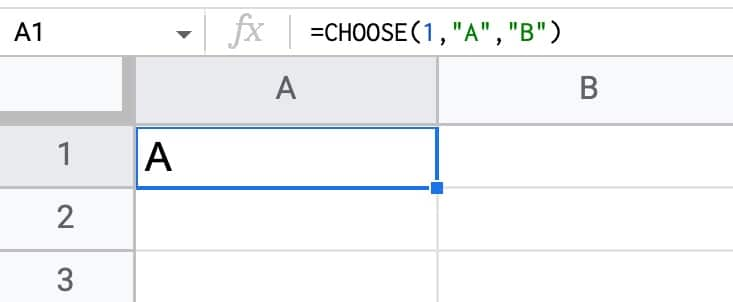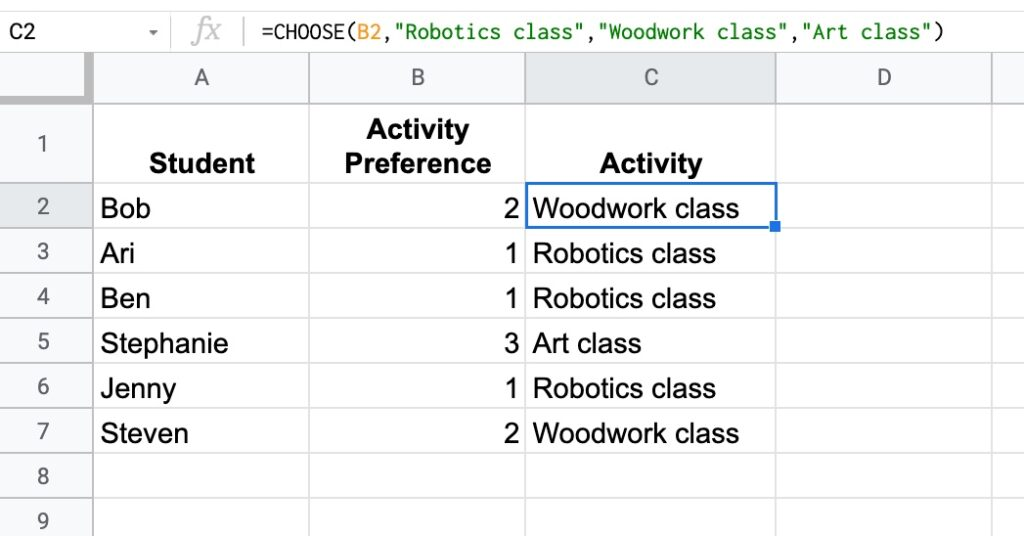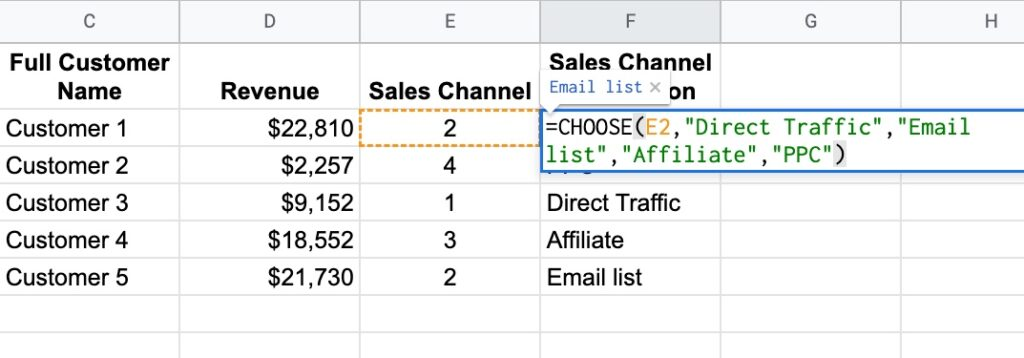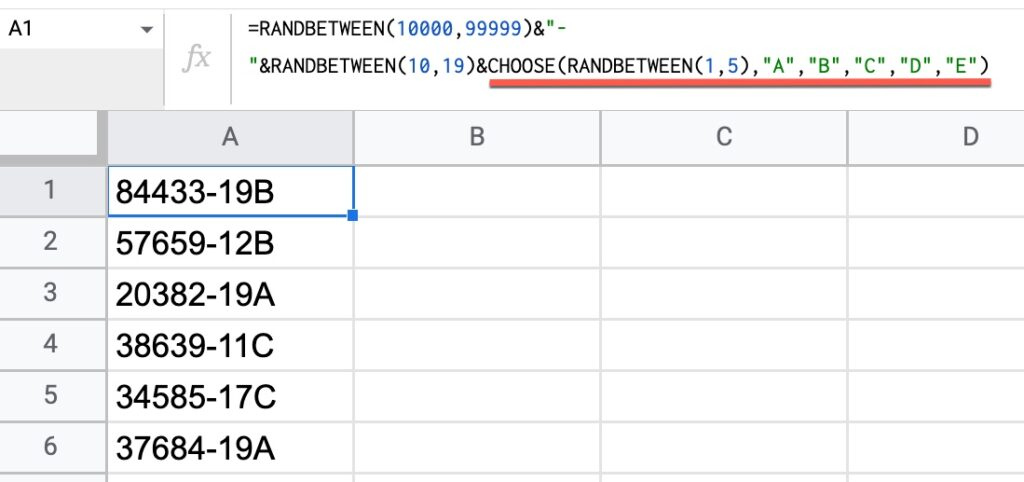# How To Use The Choose Function In Google Sheets

The CHOOSE Function in Google Sheets lets you choose between different options.

It’s a lookup function, akin to a limited VLOOKUP rather than an alternative to the IF function.

It takes an index number and returns a value at that numbered position from the list of possible options.

Here’s a simple example:

`=CHOOSE(1,"A","B")`

which will output:The first argument is the index number: 1.

Subsequent arguments are possible choices. The CHOOSE function returns the value at index position 1 in this case, i.e. “A”.

If I changed the index number to 2, the CHOOSE function would output “B”.

🔗 Get this example and others in the template at the bottom of this article.

## CHOOSE Function Syntax

`=CHOOSE(index, choice1, [choice2, ...])`

It takes a minimum of 2 arguments:

`index`

The first argument is required. It’s a number that indicates the position of the value you want to return from the list of possible values. It can be a number, a reference to a cell containing a number, or a nested function that generates a number.

`choice1`

The second argument is required and is the first possible value to return. Can be a value, reference to a cell, or a nested formula.

`[choice2, ...]`

Optional additional choices, up to a maximum of 29.

### CHOOSE Function Notes

The index number must lie between 1 and the number of choices (up to a maximum of 29).

If the index number is negative, 0, or greater than the number of choices, a #NUM! error is returned.

## When To Use The CHOOSE Function

The CHOOSE function can be used to lookup values when you have a limited number of them. It’s simpler and quicker than creating a VLOOKUP function, but doesn’t work so well with lots of values.

Here’s an example dataset showing students and their preferred activity: 1, 2, or 3.

The CHOOSE function quickly converts that to the activity name:

`=CHOOSE(B2,"Robotics class","Woodwork class","Art class")`In this example, we referenced cell B2 as the input.

## CHOOSE Formula Example

Suppose you have a dataset with a column of codes that represent the sale referral type.

CHOOSE converts the code to a description that we can understand:In this example, the CHOOSE formula is:

`=CHOOSE(E2,"Direct Traffic","Email list","Affiliate","PPC")`

Again, the index number is in a cell (E2) rather than hard-coded into the CHOOSE formula.

## Generating Random Data

Another great use case for CHOOSE is to generate random data.

For example, this formula will randomly select A, B, C, D, or E:

`=CHOOSE(RANDBETWEEN(1,5),"A","B","C","D","E")`

It uses the RANDBETWEEN function to generate a random number between 1 and 5 that then serves as the index number for CHOOSE.

This can be combined with other string formulas to generate fake data, e.g. this set of fictional invoice numbers:The formula to generate the data shown in this image is:

`=RANDBETWEEN(10000,99999)&"-"&RANDBETWEEN(10,19)&CHOOSE(RANDBETWEEN(1,5),"A","B","C","D","E")`

## CHOOSE Function Template

Feel free to make a copy: File > Make a copy…

If you can’t access the template, it might be because of your organization’s Google Workspace settings.

In this case, right-click the link to open it in an Incognito window to view it.

1.Oussama says: